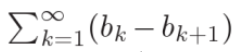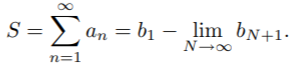# Telescoping Series

A telescoping series is any series with terms that cancel out with other terms. It’s called “telescopic” because part of each term is canceled out by a later term, collapsing the series like a folding telescope.

This type of series doesn’t have a set form like the geometric series or p-series. However, a typical way to define such a series is given by:Where bk is a sequence of real numbers.

## Sum of a Telescoping Series

Most of the terms in a telescoping series cancel out; This makes finding the sum of this type of series relatively easy. That said, there isn’t a test you can apply to find out if the series you are dealing with is telescopic. In addition, you can’t make any assumptions about which terms will cancel out, or how many. While it’s common to be left with one or two terms at the beginning or end, you may be left with three or four (Mullen, 2008).

Given that a typical telescoping series is given by an = bnbn + 1, the partial sums will have the form:

• S1 = a1 = b1b2,
• S2 = a1 + a2 = (b1b2) + (b2b3) = b1b3,
• S3 = a1 + a2 + a3= (b1b2) + (b2b3) + (b3b4)= b1b4 …,

If the sequence bn converges, then the sum of the series is (Calhoun):## Formal Definition of a Telescoping Series

Any sequence an can be expressed as a telescoping series. Having terms that cancel is definitely a hallmark of the series, but more specifically the partial sums have a finite number of terms left, after cancellation.

If an is a sequence of numbers, then a telescoping series is defined as:## References

Calhoun, D. Tips for determining convergence or divergence of an infinite series. Retrieved July 1, 2020 from: https://math.boisestate.edu/~calhoun/teaching/Previous/Math175_Spring2012/series.pdf
Ghorpade, S. et al. A Course in Calculus and Real Analysis (Undergraduate Texts in Mathematics) . Springer. 2006.
Mullen, K. Mathematics and Statistics. EDTECH. 2018.

CITE THIS AS:
Stephanie Glen. "Telescoping Series" From StatisticsHowTo.com: Elementary Statistics for the rest of us! https://www.statisticshowto.com/telescoping-series/